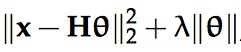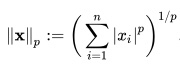# Regularized Least Squares

Regularized least squares is a way of solving least squares regression problems with an extra constraint on the solution. The constraint is called regularization.

Regularization limits the size of coefficients in the least squares method in a simple way: it adds a penalty term to the error. While doing a standard least squares regression analysis all we are doing is trying to minimize the squares of the distances from our model to each data point.

## Notation

In regularized least squares we try to minimizehere λ is greater than zero, and || · || is some penalty function– for instance, it could be a norm. θ is the parameter of our model; the parameter we are trying to find, and H is what is called the projection matrix or hat matrix, a known matrix we use just to make this analysis easier.

The norm, incidentally, is what we get if we translate our function into a vector and find the length of that vector. The p-norm (in a p dimensional space) is given byGeneralizing the concept of norm to an infinite dimensional space, the norm of any function is:## Reasons to Use Regularized Least Squares (RLS)

If regularized least squares analysis is more complicated to perform, why bother? There are two main reasons.

If there are more variables than observations in a linear system, the least squares problem (done the ordinary way) is an ill posed problem. It’s impossible to fit, because there are infinitely many solutions. With regularized least squares you can still get a uniquely determined solution.

Sometimes, even when there are not more variables than observations, the system still has a problem with generalizing. Typically this is because of what we call overlearning. Every data system will have noise, which isn’t relevant to the problem. Overlearning happens when a complex model ‘learns the noise’ as well as the focus of the analysis. Regularization, especially where the constraint is well chosen to reflect prior knowledge we might have, prevents overlearning and improves the generalizability of the problem; i.e. makes the solution we find relevant to other instances of what we’re studying.

## References

Huttunen, H. (2013). Least Squares Revisited. Retrieved January 10, 2018 from: http://www.cs.tut.fi/~hehu/SSP/lecture8.pdf
Leykekhman, D. (2008) Regularized Linear Least Squares Problems. Retrieved January 10, 2018 from: http://www.math.uconn.edu/~leykekhman/courses/MATH3795/Lectures/Lecture_10_Linear_least_squares_reg.pdf.

CITE THIS AS:
Stephanie Glen. "Regularized Least Squares" From StatisticsHowTo.com: Elementary Statistics for the rest of us! https://www.statisticshowto.com/regularized-least-squares/